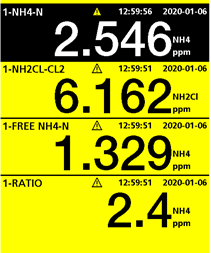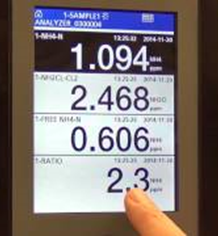# How is the ratio result on the 5500sc Ammonia Monochloramine Analyzer calculated?

## Document ID

Document ID TE12249

## Published Date

Published Date 01/23/2020
Question
How is the ratio result on the 5500sc Ammonia Monochloramine Analyzer calculated?
Summary
Explanation on how the Monochloramine to Ammonia ratio is calculated on the 5500sc AMC with examples.
The 5500sc Ammonia Monochloramine Analyzer displays four results.
• The top result is Total Ammonia as Nitrogen
• The next result is Monochloramine as Chlorine
• The next is Free Ammonia as Nitrogen
• The bottom result is the Ratio of Chlorine to Nitrogen

The ratio of Chlorine to Nitrogen is calculated by dividing the Monochloramine as Chlorine result by the Total Ammonia as Nitrogen result. This ratio is always to one as it is defined by how many parts Nitrogen there are to one part Chlorine.

Ex. 1.In this example the Monochloramine as Chlorine result is 6.162 ppm NH2Cl-Cl2 and the Total Ammonia as Nitrogen result is 2.546 ppm NH4-N
The ratio therefore is 6.162/2.546= 2.42:1 displayed as 2.4 N:Cl2

Ex. 2In this example the Monochloramine as Chlorine result is 2.468 ppm NH2Cl-Cl2 and the Total Ammonia as Nitrogen result is 1.094 ppm NH4-N
The ratio therefore is 2.468/1.094= 2.255:1 displayed as 2.3 N:Cl2